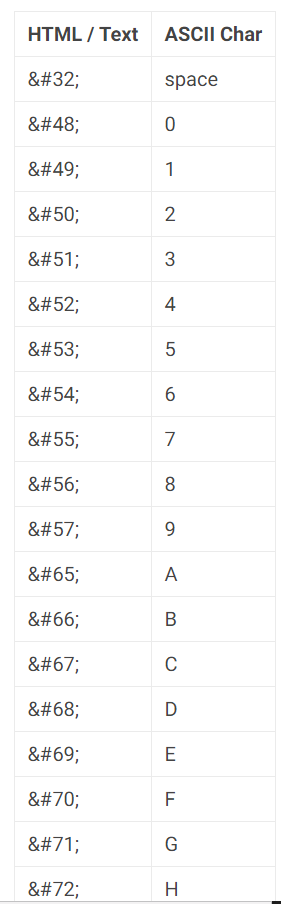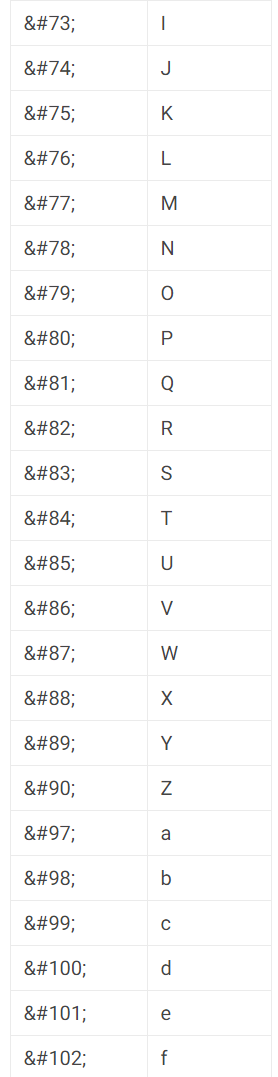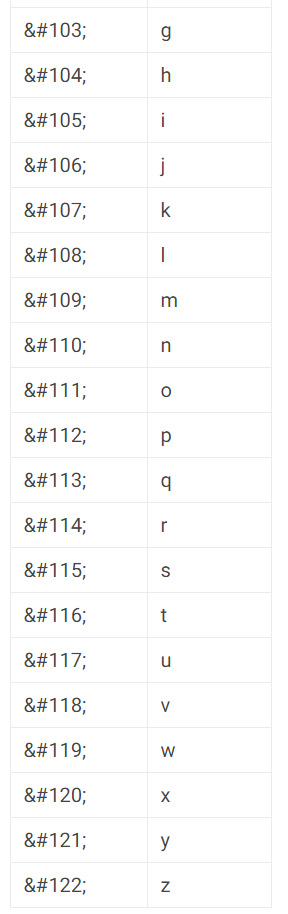# Ascii To Text

## ASCII to Text Converter

Our ASCII translator can convert ASCII strings and characters in plain text. All the applications which deal with text use ASCII numbers and as computers only take input information as numbers so that for such characters, ASCII is the best representation for which a computer can read it.

ASCII also contains code known as control characters used to change the look of the text. There are 128 characters, each character has 7 bits and is 1 bit less than 1 byte, and is allocated as numbers from 0 to 127.

## How to use our ASCII to Text converter?

This ASCII to text converter is very easy to use. Follow these steps to convert your ASCII data to plain text.

1. Enter your ASCII text in the given input box above.
2. Press the "Calculate"
3. See the output converted in plain text in the result tab.

You can also load sample data if you want by clicking on the "Load Sample Data" button.

## How to Convert ASCII to Text

Follow these steps to convert ASCII to text.

1. Get the ASCII that you want to convert to text.
2. Use the ASCII table given below to get a text character against each ASCII number.
3. Convert ASCII numbers into text characters by repeating these steps.

You can use ASCII decoder above for quick and convenient conversion.

### Example

Let's convert the " 073 032 076 111 118 101 032 067 111 110 118 101 114 115 105 111 110" ASCII string to text.

• Check the ASCII table for each character in the string "80 114 101 112 111 115 116 115 101 111." We have got all of the following from the ASCII table below.

073 = I, 032 = Space, 076 = L, 111 = o, 118 = v, 101 = e, 032 = Space, 067 = C, 111 = o, 110 = n, 118 = v, 101 = e, 114 = r, 115 = s, 105 = i, 111 = o, 110 = n

• Use these characters to form a text string.

The above ASCII string is equal to “I Love Conversion.”

## How to convert ASCII character ‘65’ to Text?

1. Get the ASCII table or refer to the table given below.
2. Locate the character '65' in the table.
3. Locate the corresponding character in the Html or text column, which is the capital 'A.'
4. So, '65' is equal to capital 'A' in the plain text.

## How to convert '72, 101, 108, 108, 111' to Text?

1. Get the ASCII table or check the table given below.
2. Identify each character in ASCII string '72, 101, 108, 108, 111' in the
3. Identify the corresponding characters in the text column one by one and write down.
4. ASCII string '72, 101, 108, 108, 111' is equal to "Hello."

You can also use our Text to ASCII converter, Binary to Decimal, ASCII to Decimal, or ASCII to Hex converter.

## ASCII to Text table

You can find the ASCII to text table below.#### Need Custom Calculator?

With Over Online Tools, eCalculator.co Helping Millions of Students, Teachers, Businessmen & Nutritionists Every Month.